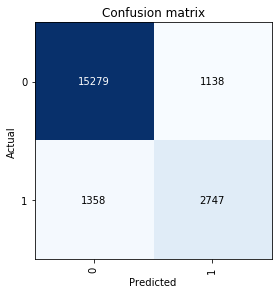# [Tabular] Very High Loss & Okay Accuracy

205205 rows × 495 columns
Data setup:

``````data = TabularDataBunch.from_df(".", df, dep_var="target", bs=1000,
cont_names=cont_vars, cat_names=cat_vars,
valid_idx=valid_idx, procs=[FillMissing, Categorify, Normalize])
``````

Learner setup:

``````emb_sizes = {x:10 for x in cat_vars}
learn = tabular_learner(data, layers=[200, 100], emb_drop=0.04, emb_szs=emb_sizes,
ps=[0.001,0.01], metrics=accuracy)
learn.lr_find()
learn.recorder.plot()
learn.fit_one_cycle(15, 1e-2, wd=0.01)
``````

I also tried with these learning rates: 1e-3, 1e-2 and, 5e-3.

what is weird: loss is very high, while accuracy is reasonable.What I have investigated:
learn.loss_func returns:
`FlattenedLoss of CrossEntropyLoss()`

Is the model very naive and always predicting 0?
No; the validation set is 80% zeros and 20% ones. Also here is the CM:Is the architecture too big or too small?
I tried [100, 500], [10, 100, 10], [10, 10, 10], [10, 10], , [1000, 100]
All yielded very similar results, which is weird, isn’t it?

I tried with and without weight decay of 0.01, with and without emb_drop of 0.1 and 0.04, with and without ps=[0.001,0.01]

A random forests model had an accuracy of 87~88% and a boosted one reached 89% with close confusions matrices to what’s shown above.

What do you think is going on here?

When you do your preprocessing, are you essentially recreating the same encoding setup as what fastai does? Are you keeping track of if values are missing? (fastai does something special here with that0.

1 Like

Thanks a lot for your response Mueller.
What I was doing was replacing missing values with -1 and not adding an extra column.
Anyway I discarded that and started over with a copy of the dataframe without any processing done to its categories. And I am relying on fastai now to do all the processing as follows:

``````data = TabularDataBunch.from_df(".", df, dep_var="target", bs=1000,
cont_names=cont_vars, cat_names=cat_vars,
valid_idx=valid_idx, procs=[FillMissing, Categorify, Normalize])
``````

here is the model setup:

``````learn = tabular_learner(data, layers=[100, 500], emb_drop=0.04,
ps=[0.001,0.01], metrics=accuracy)
learn.lr_find()
learn.recorder.plot()
learn.fit_one_cycle(5, 3e-3, wd=0.01)
``````

And the results are still the same:

I did the following:

``````preds, ans = learn.get_preds(ds_type=DatasetType.Valid)
learn.loss_func(preds, ans)
``````

and it returned

tensor(0.4638)

How come!